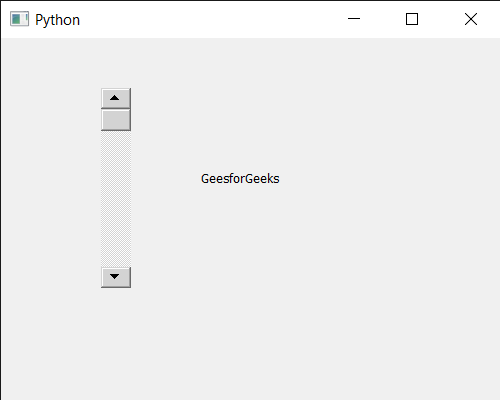GFG App
Open AppBrowser
Continue

# PyQt5 QScrollBar – Setting Window Opacity property

In this article we will see how we can set window opacity property to the QScrollBar. QScrollBar is a control that enables the user to access parts of a document that is larger than the widget used to display it. Slider is the scroll-able object inside the bar. This property holds the level of opacity for the window. The valid range of opacity is from 1.0 (completely opaque) to 0.0 (completely transparent).

Note : On X11 you need to have a composite manager running, and the X11 specific _NET_WM_WINDOW_OPACITY atom needs to be supported by the window manager you are using.

In order to do this we will use `setWindowOpacity` method with the scroll bar object.

Syntax : scroll.setWindowOpacity(n)

Argument : It takes float as argument

Return : It returns None

Below is the implementation

 `# importing libraries ` `from` `PyQt5.QtWidgets ``import` `*`  `from` `PyQt5 ``import` `QtCore, QtGui ` `from` `PyQt5.QtGui ``import` `*`  `from` `PyQt5.QtCore ``import` `*`  `import` `sys ` ` `  ` `  `class` `Window(QMainWindow): ` ` `  `    ``def` `__init__(``self``): ` `        ``super``().__init__() ` ` `  `        ``# setting title ` `        ``self``.setWindowTitle(``"Python "``) ` ` `  `        ``# setting geometry ` `        ``self``.setGeometry(``100``, ``100``, ``500``, ``400``) ` ` `  `        ``# calling method ` `        ``self``.UiComponents() ` ` `  `        ``# showing all the widgets ` `        ``self``.show() ` ` `  ` `  ` `  `    ``# method for components ` `    ``def` `UiComponents(``self``): ` ` `  `        ``scroll ``=` `QScrollBar(``self``) ` ` `  `        ``# setting geometry of the scroll bar ` `        ``scroll.setGeometry(``100``, ``50``, ``30``, ``200``) ` ` `  `        ``# making its background color to green ` `        ``scroll.setStyleSheet(``"background : lightgrey;"``) ` ` `  `        ``# setting window opacity property ` `        ``scroll.setWindowOpacity(``1.0``) ` ` `  `        ``# creating a label ` `        ``label ``=` `QLabel(``"GeesforGeeks"``, ``self``) ` ` `  `        ``# setting geometry to the label ` `        ``label.setGeometry(``200``, ``100``, ``300``, ``80``) ` ` `  `        ``# making label multi line ` `        ``label.setWordWrap(``True``) ` ` `  `        ``# getting value changed signal ` `        ``scroll.valueChanged.connect(``lambda``: do_action()) ` ` `  `        ``# method called when signal is emitted ` `        ``def` `do_action(): ` `             `  `            ``# setting text to the label ` `            ``label.setText(``"Current Value : "` `+` `str``(scroll.value())) ` ` `  ` `  ` `  `# create pyqt5 app ` `App ``=` `QApplication(sys.argv) ` ` `  `# create the instance of our Window ` `window ``=` `Window() ` ` `  `# start the app ` `sys.exit(App.``exec``()) `

Output :My Personal Notes arrow_drop_up